Homework Help Question & Answers

# ignore the top part. need the expected return for stock C and stock T. also the...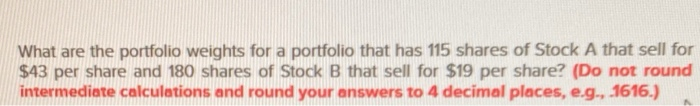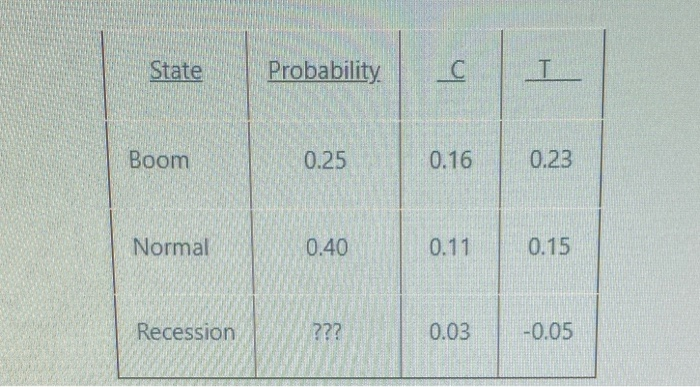ignore the top part. need the expected return for stock C and stock T. also the variance for stock C and standard deviation for stock T. thanks
What are the portfolio weights for a portfolio that has 115 shares of Stock A that sell for $43 per share and 180 shares of Stock B that sell for$19 per share? (Do not round intermediate calculations and round your answers to 4 decimal places, e.g., .1616.)
State Probability Boom 0.25 0.16 0.23 Normal 0.40 0.11 0.15 Recession ??? 0.03 -0.05

#### Homework Answers

Answer #1

The probability of total state is 1. Thus, probability of Recession is = 1 - probability of Boom - probability of Normal

= 1 - 0.25 - 0.40

= 0.35

Calculating the Expected Return, Variance and Standard Deviation:-

 Prob (P) Stock Return (C) (%) Stock Return (T) (%) (P)*(C) (P)*(T) Deviation        [R-E(C)] Deviation        [R-E(T)] [R-E(C)]^2 {[R-E(C)]^2}*(P) [R-E(T)]^2 {[R-E(T)]^2}*(P) 0.25 0.1600 0.2300 0.0400 0.0575 0.0655 0.1125 0.0043 0.0011 0.0127 0.0032 0.40 0.1100 0.1500 0.0440 0.0600 0.0155 0.0325 0.0002 0.0001 0.0011 0.0004 0.35 0.0300 -0.0500 0.0105 -0.0175 -0.0645 -0.1675 0.0042 0.0015 0.0281 0.0098 0.09 0.12 0.0026 0.0134

- Expected return for Stock C =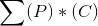= 0.09

- Expected return for Stock T =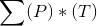= 0.12

- Variance for Stock C =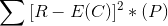= 0.0026

- Standard Deviation for Stock T =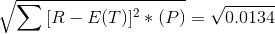= 0.1158

Know the answer?
Your Answer:

#### Post as a guest

Your Name:

What's your source?

#### Earn Coin

Coins can be redeemed for fabulous gifts.

Not the answer you're looking for? Ask your own homework help question. Our experts will answer your question WITHIN MINUTES for Free.
Similar Homework Help Questions
• ### What are the portfolio weights for a portfolio that has 122 shares of Stock A that...

What are the portfolio weights for a portfolio that has 122 shares of Stock A that sell for $32 per share and 102 shares of Stock B that sell for$22 per share? (Do not round intermediate calculations and round your answers to 4 decimal places, e.g., .1616.)

• ### What are the portfolio weights for a portfolio that has 125 shares of Stock A that...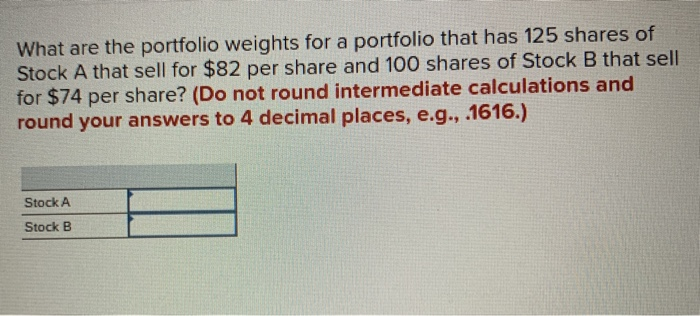What are the portfolio weights for a portfolio that has 125 shares of Stock A that sell for $82 per share and 100 shares of Stock B that sell for$74 per share? (Do not round intermediate calculations and round your answers to 4 decimal places, e.g., .1616.) Stock A Stock B

• ### Rate of Return if State Occurs State of Economy State of Economy Stock A Stock B...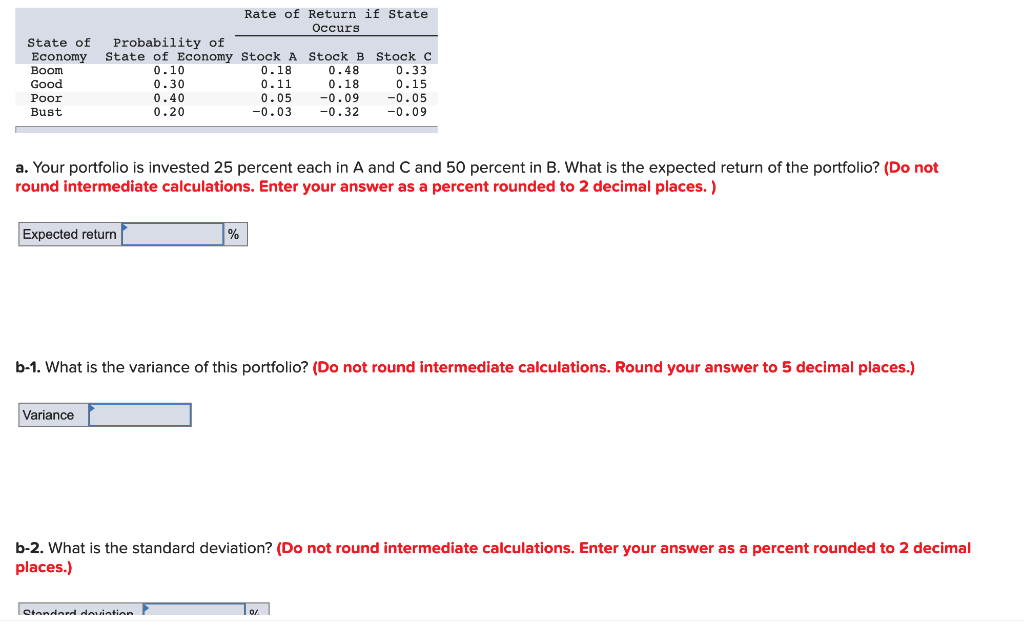Rate of Return if State Occurs State of Economy State of Economy Stock A Stock B Stock C Boom Probability of 0.18 0.11 0.48 0.18 -0.09 0.32 0.33 0.15 0.10 0.30 0.40 Good -0.05 -0.09 0.05 -0.03 Poor 0.20 Bust a. Your portfolio is invested 25 percent each in A and C and 50 percent in B. What is the expected return of the portfolio? (Do not round intermediate calculations. Enter your answer as a percent rounded to 2 decimal...

• ### Rate of Return if State Occurs State of Probability of Economy State of Economy Stock A...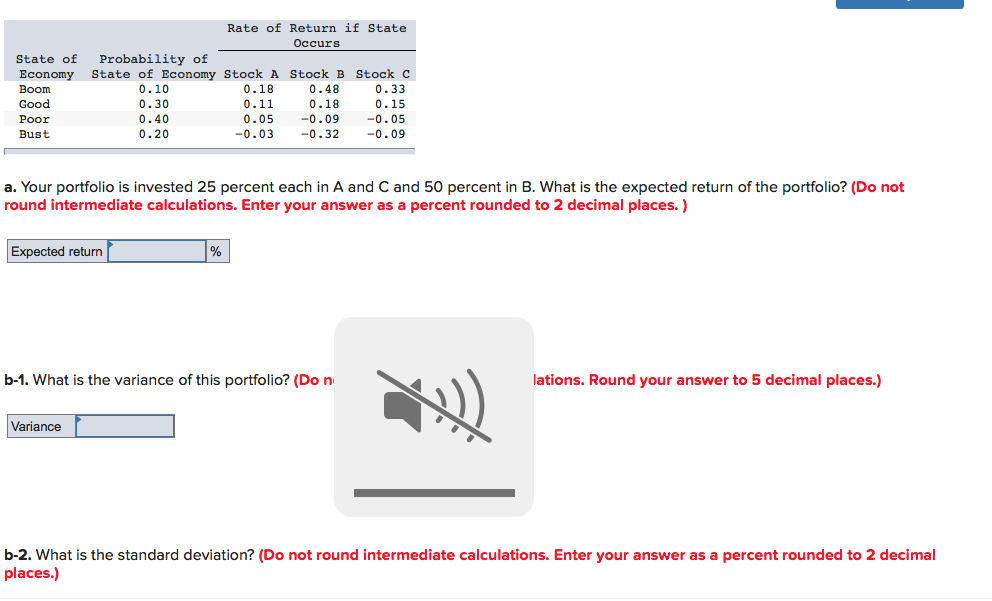Rate of Return if State Occurs State of Probability of Economy State of Economy Stock A Stock B Stock C Boom 0.10 0.18 0.48 0.33 Good 0.30 0.11 0.18 0.15 Poor 0.40 0.05 -0.09 -0.05 Bust 0.20 -0.03 -0.32 -0.09 a. Your portfolio is invested 25 percent each in A and C and 50 percent in B. What is the expected return of the portfolio? (Do not round intermediate calculations. Enter your answer as a percent rounded to 2 decimal...

• ### What are the portfolio weights for a portfolio that has 135 shares of Stock A that...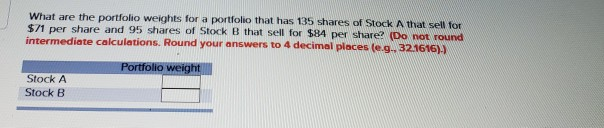What are the portfolio weights for a portfolio that has 135 shares of Stock A that sell for $71 per share and 95 shares of Stock B that sell for$84 per share? (Do not found intermediate calculations. Round your answers to 4 decimal places (e... 32.1616).) Portfolio weight Stock A Stock B Consider the following information. Rate of Return if State Occurs Probability of State of State Economy of Economy Recession 20 Normal Boom Book 50 Print Required: Calculate...

• ### Please answer all 3 questions State of Economy Boom Good Poor Bust Rate of Return if...Please answer all 3 questions State of Economy Boom Good Poor Bust Rate of Return if State Occurs Probability of State of Economy Stock A Stock B Stock C 0.10 0.18 0.48 0.33 0.30 0.11 0.18 0.15 0.40 0.05 -0.09 -0.05 0.20 -0.03 -0.32 -0.09 a. Your portfolio is invested 25 percent each in A and C and 50 percent in B. What is the expected return of the portfolio? (Do not round intermediate calculations. Enter your answer as a...

• ### Consider the following information: Rate of Return if State Occurs State of Probability of Stock Stock...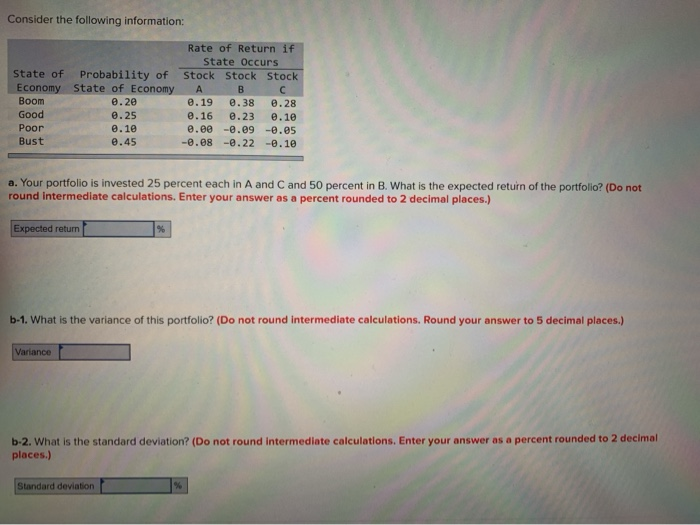Consider the following information: Rate of Return if State Occurs State of Probability of Stock Stock Stock Economy State of Economy A B C Boom 0.28 0.19 0.38 0.28 Good 0.25 0.16 0.23 0.10 Poor 0.10 e.ee -.09 -0.05 Bust 0.45 -0.08 -0.22 -0.10 a. Your portfolio is invested 25 percent each in A and C and 50 percent in B. What is the expected return of the portfolio? (Do not round Intermediate calculations. Enter your answer as a percent...

• ### Check my work Consider the following information: 10 points Rate of Return if State Occurs State...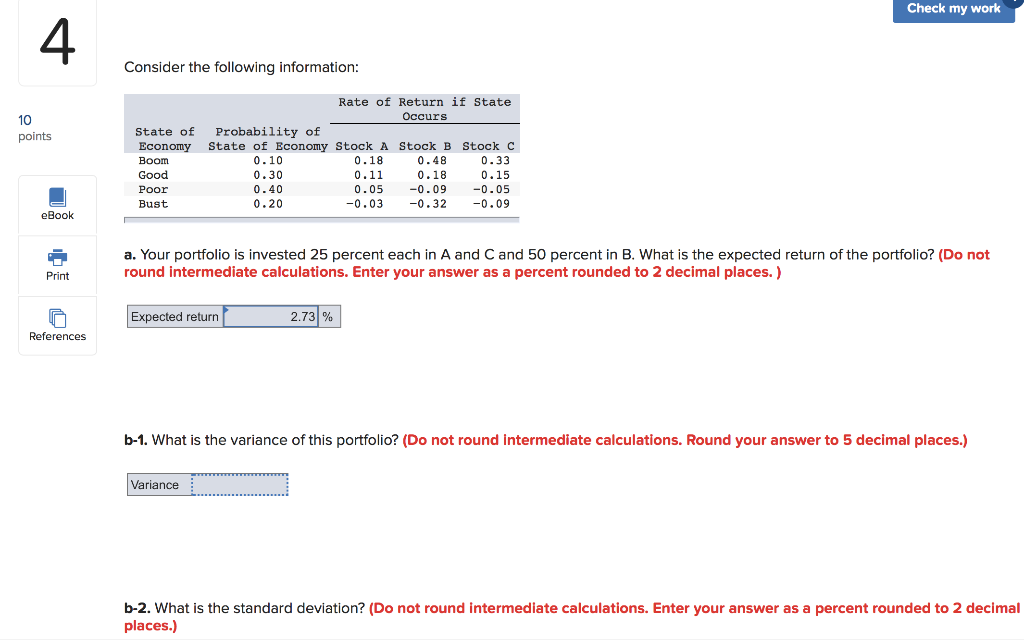Check my work Consider the following information: 10 points Rate of Return if State Occurs State of Probability of Economy State of Economy Stock A Stock B Stock C Boom 0.10 0.18 0.48 0.33 Good 0.30 0.11 0.18 0.15 Poor 0.40 0.05 -0.09 -0.05 Bust 0.20 -0.03 -0.32 -0.09 eBook a. Your portfolio is invested 25 percent each in A and C and 50 percent in B. What is the expected return of the portfolio? (Do not round intermediate calculations....

• ### PART III RISK AND RETURN Cell for "2" State of the Economy Worst case Poor case...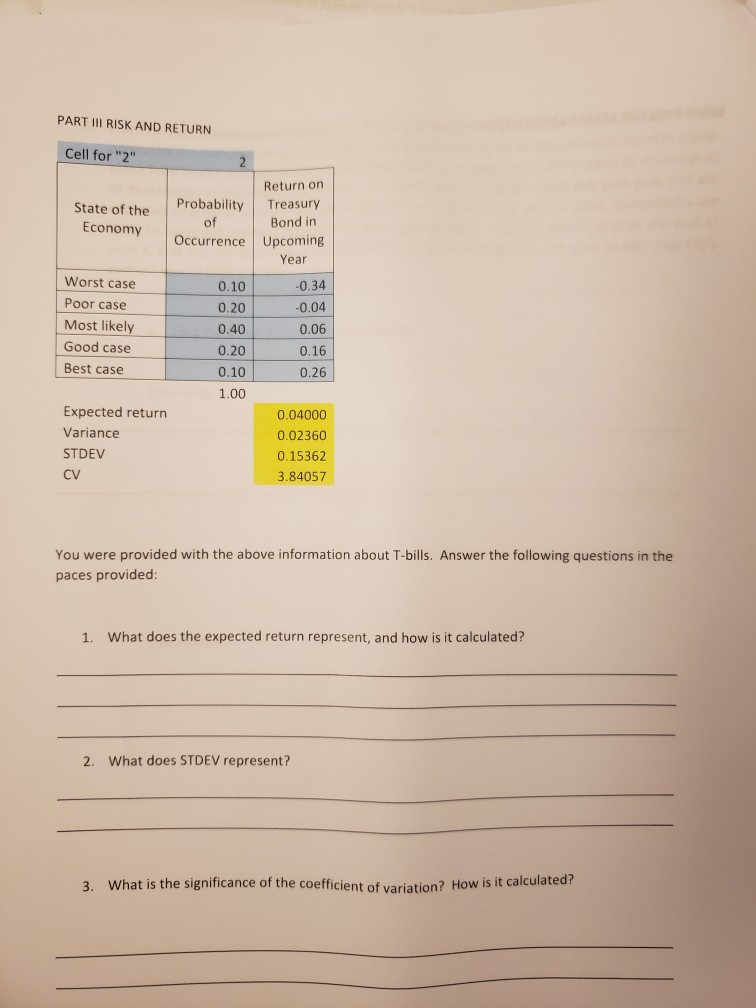PART III RISK AND RETURN Cell for "2" State of the Economy Worst case Poor case Most likely Good case Best case Return on Probability Treasury of Bond in Occurrence Upcoming Year 0.10 -0.34 0.20 -0.04 0.40 0.06 0.20 0.16 0.10 0.26 1.00 0.04000 0.02360 0.15362 3.84057 Expected return Variance STDEV CV You were provided with the above information about T-bills. Answer the following questions in the paces provided: 1. What does the expected return represent, and how is it...

Free Homework App

Scan Your Homework
to Get Instant Free Answers
Need Online Homework Help?

Get Answers For Free
Most questions answered within 3 hours.